Next: Output zero case: Up: Division Previous: Division

#### Modification of mantissas

We first need to modify the mantissas x and y' of the numerator and denominator in such a way as to allow us to perform division on the streams. Using the naïve approach of simply using the mantissas directly this would be impossible: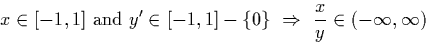However we can use shift operations and the identities from section 3.3.1 to find an exponent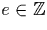and a numeral y' such that: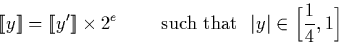Essentially we insist that the stream y starts with a non-zero digit, and that the first two digits are not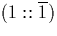or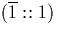. Now we can say: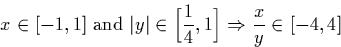The algorithm will now compute. For simplicity we now assume that y always starts with the digit one. In order to compute division by a negative y, it is sufficient to negate y to make it positive, and then negate either the numerator x or the result of the whole division. Hence from now on: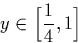We describe the algorithm and prove its correctness by examining cases. We look at all possible combinations of digits at the head of the input streams, and for each case show how we can find a dyadic digit which we can output, and express the remainder of the result as a recursive call to the division algorithm.

The algorithm is broken up into the cases which produce the digit zero as output, those which produce a positive digit, and those which produce a negative digit.Next: Output zero case: Up: Division Previous: Division
Martin Escardo
5/11/2000